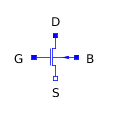Cone - MapleSim Help

Cone

Cone rigid bodyDescription The Cone component models a homogeneous conical rigid body along a given axis with a predefined density. Based on the properties, i.e., axial unit vector, length, radius, and density, the center of mass, total mass, and moments of inertia are calculated for this rigid body.Connections

 Name Description Modelica ID $\mathrm{frame__a}$ Coordinate on one end of the cone axis frame_a $\mathrm{frame__b}$ Coordinate on the other end of the cone axis frame_b $\mathrm{frame__c}\left[n\right]$ An array of additional frames on the cone axis frame_c[n]Parameters

 Name Default Units Description Modelica ID $\mathrm{e__axis}$ $\left[1,0,0\right]$ Axial unit vector e_axis L $1$ m Cone length L $\mathrm{R__a}$ $0.4$ $m$ Cone radius at frame_a Ra $\mathrm{R__b}$ 0.1 m Cone radius at frame_b Rb $\mathrm{R__ai}$ $0$ $m$ Cone inner radius at frame_a Rai $\mathrm{R__bi}$ 0 m Cone inner radius at frame_b Rbi Select density User defined Select a predefined material density selectDensity $\mathrm{ρ}$ 1000 $\frac{\mathrm{kg}}{{m}^{3}}$ Cylinder user-defined material density customDensity Use additional frames false True means additional frames can be added addFrames $\mathrm{L__add}$ $\left[\frac{L}{2}\right]$ m Each value in this array defines a frame on the cylinder axis w.r.t. frame_a L_add[:] Use initial conditions false True means parameters for specifying initial conditions for frame_a are enabled. Refer to: Rigid Body useICs Show visualization true True means the cylinder geometry is visible in the 3-D playback visualization Transparent false True means the geometry is transparent in the 3-D playback transparent ColorCylinder color in the 3-D playback colorEquations The two end frames of the cone have the same orientation. The translation vectors  and  w.r.t. frame_a define the frame_b and the center of mass frame, respectively. Center of mass location is calculated as $\mathrm{L__com}=\frac{L\left({\mathrm{R__a}}^{2}+2\mathrm{R__a}\mathrm{R__b}-{\mathrm{R__ai}}^{2}-2\mathrm{R__ai}\mathrm{R__bi}+3{\mathrm{R__b}}^{2}-3{\mathrm{R__bi}}^{2}\right)}{4{\mathrm{R__a}}^{2}+4\mathrm{R__a}\mathrm{R__b}-4{\mathrm{R__ai}}^{2}-4\mathrm{R__ai}\mathrm{R__bi}+4{\mathrm{R__b}}^{2}-4{\mathrm{R__bi}}^{2}}$ Cone mass is calculated as where the cone material density, ρ, can be defined using the "Select density" parameter. This parameter lets the user either enter a value or select among predefined material densities.Figure 1: Different options for the "Select density" property   Assuming the default direction of $\left[1,0,0\right]$ for the cylinder axis, the moments of inertia expressed from the center of mass frame are   The right-hand side of these equations will interchange if another axial unit vector is specified.ExamplesSwinging T-Shaped Object

Figure 2 shows the layout of a MapleSim model that uses two Cone components to simulate a freely swinging T-shaped object. Note how the frame_a of the vertical cylinder (axis = [0,1,0]) is connected to the frame_c of the horizontal cylinder (axis=[1,0,0]) to form the T-shaped object. For the horizontal cone, frame_c is located halfway $\left(\mathrm{L__add}=\left[\frac{L}{2}\right]\right)$ between frame_a and frame_b. A snapshot of the 3-D playback is shown in Figure 3.Figure 2: Model layout Figure 3: 3-D playback snapshot